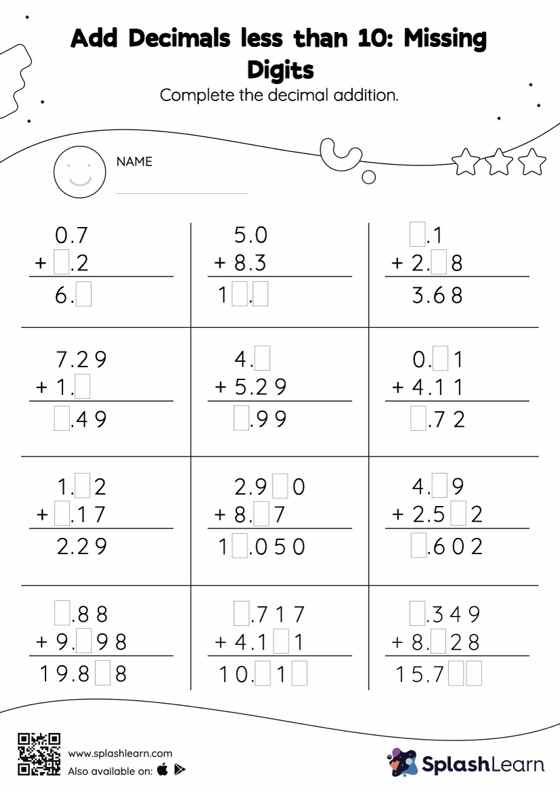# Add Decimals less than 10: Missing Digits Worksheet

Home > Add Decimals less than 10: Missing DigitsThis worksheet gives students an opportunity to apply the concepts of addition to add decimals less than 10. In this worksheet, students add decimals by aligning the decimal points and using zero as a placeholder. They then use the relationship between addition and subtraction to find the missing number in the add decimals less than 10 worksheet. In this worksheet, students practice solving problems using the column method. This method is especially helpful with problems involving bigger multi-digit numbers as the format provides an easy structure to follow.